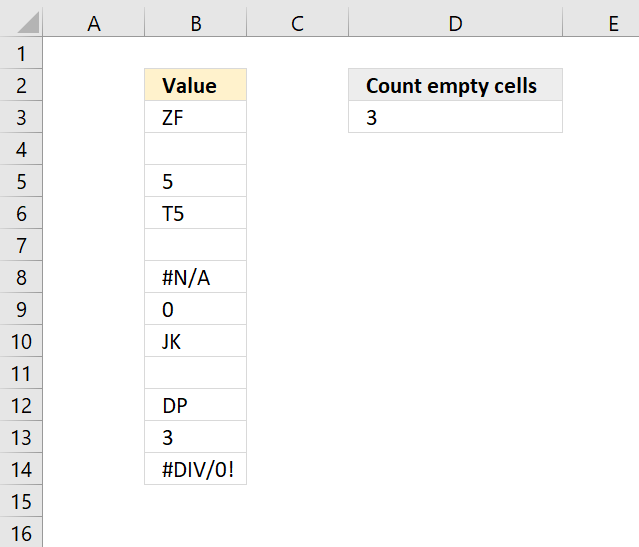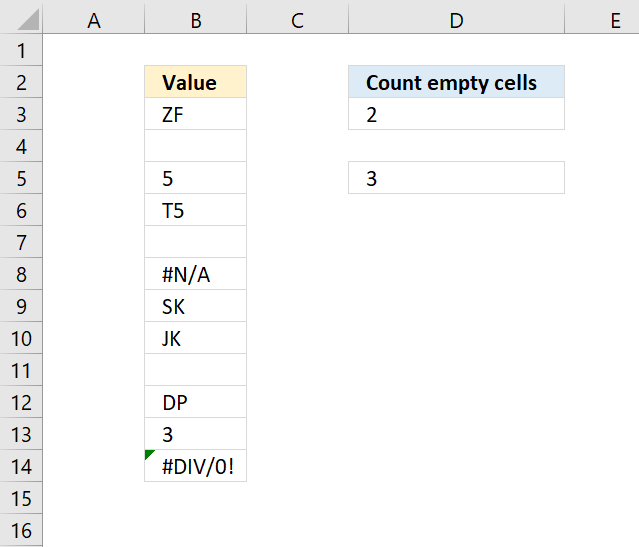Author: Oscar Cronquist Article last updated on November 06, 2018The image above shows the COUNTBLANK function counting empty cells in cell range B3:B14. Note that the COUNTBLANK function ignores error values, most Excel functions don't.

Formula in cell D3:

=COUNTBLANK(B3:B14)

What will this formula return if there was a space character in cell B7? The cell is not empty anymore, however, it looks empty. See image below.The following formula counts blank cells even if the cell contains one or more space characters.

Array formula in cell D5:

=SUM((IFERROR(TRIM(B3:B14),"A")="")*1)

To enter an array formula, type the formula in a cell then press and hold CTRL + SHIFT simultaneously, now press Enter once. Release all keys.

The formula bar now shows the formula with a beginning and ending curly bracket telling you that you entered the formula successfully. Don't enter the curly brackets yourself.

### Explaining formula in cell D5

#### Step 1 - Remove leading and trailing space characters

The TRIM function deletes leading and trailing space characters from a cell value, however, here I am using a cell range so we need to enter this formula as an array formula.

TRIM(B3:B14)

becomes

TRIM({"ZF";"";"5";"T5";" ";#N/A;"SK";"JK";"";"DP";"3";#DIV/0!})

and returns

{"ZF";"";"5";"T5";"";#N/A;"SK";"JK";"";"DP";"3";#DIV/0!}

#### Step 2 - Convert error values to a valid value

Also, the TRIM function doesn't ignore error values, we need to trap those error values before counting them and the IFERROR function allows you to do that.

becomes

IFERROR({"ZF";"";"5";"T5";"";#N/A;"SK";"JK";"";"DP";"3";#DIV/0!},"A")

and returns {"ZF";"";"5";"T5";"";#N/A;"SK";"JK";"";"DP";"3";#DIV/0!}.

I have entered the array in column A.

#### Step 3 - Compare each value in the array to nothing

The equal sign lets you compare the values in the array to a given condition, in this case "" is nothing.

becomes

{"ZF";"";"5";"T5";"";"A";"SK";"JK";"";"DP";"3";"A"}=""

and returns {FALSE; TRUE; FALSE; FALSE; TRUE; FALSE; FALSE; FALSE; TRUE; FALSE; FALSE; FALSE}.

I have entered the array in column A.

#### Step 4 - Convert boolean values

The SUM function can't add boolean values in an array, we need to convert them. TRUE becomes 1 and FALSE becomes 0 (zero).

becomes

({FALSE; TRUE; FALSE; FALSE; TRUE; FALSE; FALSE; FALSE; TRUE; FALSE; FALSE; FALSE})*1

and returns {0; 1; 0; 0; 1; 0; 0; 0; 1; 0; 0; 0}.

I have entered the array in column A.

#### Step 5 - Sum values

SUM((IFERROR(TRIM(B3:B14),"A")="")*1)

becomes

SUM({0; 1; 0; 0; 1; 0; 0; 0; 1; 0; 0; 0}) and returns 3 in cell D5.